# ML Aggarwal Class 10 Solutions for ICSE Maths Chapter 7 Ratio and Proportion MCQS

## ML Aggarwal Class 10 Solutions for ICSE Maths Chapter 7 Ratio and Proportion MCQS

ML Aggarwal Class 10 Solutions for ICSE Maths Chapter 7 Ratio and Proportion MCQS

Choose the correct answer from the given options (1 to 10):

Question 1.
The ratio of 45 minutes to $$5 \frac { 3 }{ 4 }$$ hours is
(a) 180 : 23
(b) 3 : 23
(c) 23 : 3
(d) 6 : 23
Solution: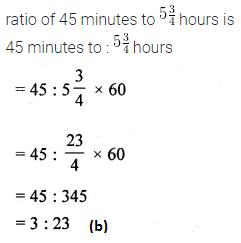Question 2.
The ratio of 4 litres to 900 mL is
(a) 4 : 9
(b) 40 : 9
(c) 9 : 40
(d) 20 : 9
Solution: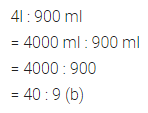Question 3.
When the number 210 is increased in the ratio 5 : 7, the new number is
(a) 150
(b) 180
(c) 294
(d) 420
Solution: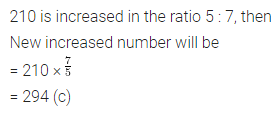Question 4.
Two numbers are in the ratio 7 : 9. If the sum of the numbers is 288, then the smaller number is
(a) 126
(b) 162
(c) 112
(d) 144
Solution: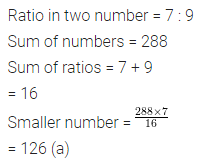Question 5.
A ratio equivalent to the ratio $$\\ \frac { 2 }{ 3 }$$ : $$\\ \frac { 5 }{ 7 }$$ is
(a) 4 : 6
(b) 5 : 7
(c) 15 : 14
(d) 14 : 15
Solution:Question 6.
The ratio of the number of edges of a cube to the number of its faces is
(a) 2 : 1
(b) 1 : 2
(c) 3 : 8
(d) 8 : 3
Solution:Question 7.
If x, 12, 8 and 32 are in proportion, then the value of x is
(a) 6
(b) 4
(c) 3
(d) 2
Solution:Question 8.
The fourth proportional to 3, 4, 5 is
(a) 6
(b) $$\\ \frac { 20 }{ 3 }$$
(c) $$\\ \frac { 15 }{ 4 }$$
(d) $$\\ \frac { 12 }{ 5 }$$
Solution:Question 9.
The third proportional to $$6 \frac { 1 }{ 4 }$$ and 5 is
(a) 4
(b) $$8 \frac { 1 }{ 2 }$$
(c) 3
(d) none of these
Solution: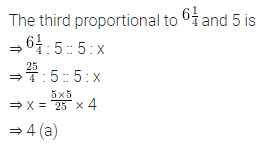Question 10.
The mean proportional between $$\\ \frac { 1 }{ 2 }$$ and 128 is
(a) 64
(b) 32
(c) 16
(d) 8
Solution: